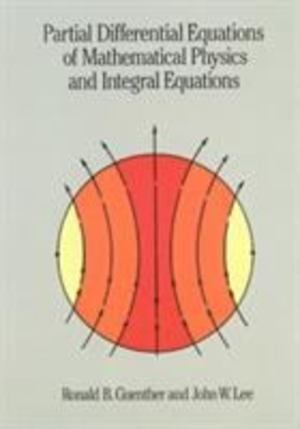# libib

## Partial Differential Equations of Mathematical Physics and Integral EquationsRonald B. Guenther, John W. Lee
This book was written to help mathematics students and those in the physical sciences learn modern mathematical techniques for setting up and analyzing problems. The mathematics used is rigorous, but not overwhelming, while the authors carefully model physical situations, emphasizing feedback among a beginning model, physical experiments, mathematical predictions, and the subsequent refinement and reevaluation of the physical model itself. Chapter 1 begins with a discussion of various physical problems and equations that play a central role in applications. The following chapters take up the theory of partial differential equations, including detailed discussions of uniqueness, existence, and continuous dependence questions, as well as techniques for constructing conclusions. Specifically, Chapters 2 through 6 deal with problems in one spatial dimension. Chapter 7 is a detailed introduction to the theory of integral equations; then Chapters 8 through 12 treat problems in more spatial variables. Each chapter begins with a discussion of problems that can be treated by elementary means, such as separation of variables or integral transforms, and which lead to explicit, analytical representations of solutions. The minimal mathematical prerequisites for a good grasp of the material in this book are a course in advanced calculus, or an advanced course in science or engineering, and a basic exposure to matrix methods. Students of mathematics, physics, engineering, and other disciplines will find here an excellent guide to mathematical problem-solving techniques with a broad range of applications. For this edition the authors have provided a new section of Solutions and Hints to selected Problems. Suggestions for further reading complete the text.

No reviews
Item Posts
No posts